• 对于许多研究人员和审查员来说，确定股票价格专业性一直是一项麻烦任务。 事实上，金融专家对股票价值预测检查领域非常感兴趣。 对于体面而有用投机，众多投机者对股市未来走势了如指掌。 强大而强大股票...
• 其次，通过基于LSTM的时间序列分类算法，进行模型训练。最后对抓取网络数据流进行入侵检测，对超过6万节点和300万条边拓扑图进行测试。最终实验结果表明，该算法具有更高准确率和召回率，可以有效地检测出...
• 防法/过程」提出一种基于机器学习算法的研究热点趋势预测方法与分析框架，以基因工程领域为例利用主题概率模型识别WOS核心集中论文摘要数据研究热点主题并进行主题演化关联构建，然后选取BP神经网络、支持向量机及...
• 基于改进萤火虫算法的LSTM预测模型，韩宪斌，亓峰，本文针对LSTM神经网络预测时存在的收敛慢、超参数调整困难等缺陷，提出了通过萤火虫算法优化神经网络结构以提高流量预测性能的模��计算机应用技术
• 基于Spatio-LSTM模型城市交通路网流量预测算法，高尚，李静林，在城市环境中，道路交通流量变化快速且受随机扰动影响强烈，给交通管理和通信资源分配带来诸多困难。针对交通流量预测问题，本文车流量预测
• 近年来，机器学习技术发展迅速，已经在视觉图像等领域取得很大突破，同时，也有越来越多人着眼使用机器学习方法研究金融领域问题，本文主要基于机器学习算法对金融时间序列进行预测分析，具体步骤为，先对期货...
转自（https://www.joinquant.com/view/community/detail/25917）
近年来，机器学习技术发展迅速，已经在视觉图像等领域取得很大突破，同时，也有越来越多的人着眼使用机器学习方法研究金融领域的问题，本文主要基于机器学习算法对金融时间序列进行预测分析，具体步骤为，先对期货主力合约价格数据使用去噪自编码器过滤掉随机噪声波动，再用一维卷积对滤波后的数据降维，提取主要信息，再将滤波降噪后的价格数据输入到LSTM神经网络，之后将LSTM网络的输出作为全连接网络的输入，使用交叉验证的方法训练出一个可以捕捉期货价格大幅波动前的特征的模型，再通过这个模型在实时价格序列上预测价格大幅波动的可能性以及波动的方向，接下来在预测准确率较高的时刻下单交易。
本文的实证结果表明使用自编码器和卷积对金融数据滤波降噪，再结合LSTM神经网络的模型具有一定的预测效果，证明了机器学习算法确实可以发掘金融序列的内在特征规律。
模型训练数据来自jqdata
一.模型的建立
1.1 模型框架
模型的框架是这样的：获取数据后，先使用去噪自编码器滤去数据中的噪音干扰，再用卷积提取数据中的特征，由于提取出的特征序列长度较长，之后用池化操作对提取出的特征数据降维，由于原始数据序列是一个时间序列，具有时序特征，因此将降维后的数据输入LSTM神经网络提取其中的时序特征，之后LSTM神经网络会输出一组综合了前面各网络层提取到的信息和LSTM层提取的时序特征的一个100维的特征向量，将这个100维的向量接入全连接层得到预测结果再输出。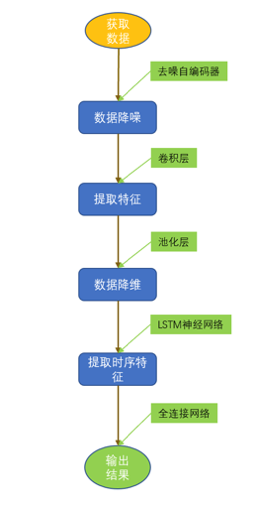1.2 为什么要用CNN-LSTM
LSTM模型的提出帮助人们更好的发现序列数据中隐含的规律，同时通过前面阅读其他学者的论文发现往往结合其他方法的LSTM模型效果会更好，再由于CNN的提出极大程度的推进了计算机视觉的发展，是一个很有效的模型。
有了卷积神经网络后，通过卷积神经网络识别图像很容易就超过了之前存在的传统图像识别算法，并且在Convolutional Neural Networks for Sentence Classification这篇论文中也指出卷积神经网络不止可以处理图像，也可以用于自然语言处理，并且从作者的实验结果上看，在数个数据集上实验效果都比较好，甚至好于之前存在的分本分类方法。也就是说卷积神经网络可以有效的用于序列分析。
1.3 数据获取
该模型主要用于主力期货价格的预测分析，本文选用的数据是螺纹钢主力期货5分钟数据，使用jqdata的API接口获取过去5年时间里的开盘价，收盘价，最高价，最低价及成交量数据用于分析，之后选出大幅度波动前的360份5分钟k线数据作为训练样本，大幅波动的方向作为训练样本的标记。
1.4 数据降噪
本文中使用去噪自编码器来滤除获取的螺纹钢期货数据中的微小噪音，使得在后面的操作中，数据更加纯净，更容易提取其中隐藏的有用特征。
自编码器是一类输入与输出相等的神经网络，结构包括编码器（encoder），解码器（decoder）两部分，在训练的时候编码器和解码器是连接在一起当作一个整体训练的，去噪自编码器是对普通自编码器的一个改进，利用普通自编码器输入等于输出的特性。
二. 交易策略
最终目的是设计用来预测大涨和大跌可能性的模型。先从数据接口下载好数据后，找到大幅涨跌之前的一段历史数据，为这些数据进行简单的标准化处理后，使用去噪自编码器滤去数据中的无用噪声，之后再用卷积核提取其中的有用特征，再将数据使用池化层处理一遍，起到压缩数据和突出重点特征的作用，等到前面这部分处理数据的过程完成后，将数据放入LSTM神经网路来找出数据中的时序特征，之后，将最终的输出结果通过全连接神经网络得到分类结果，再在模型预测上涨可能性较大的时候买多，在模型预测下跌可能性较大的时候卖空，在合适的时候平仓，反复执行此操作直到回测期结束。
2.1 具体实现细节
先获取包含开盘价，收盘价，最高价，最低价，成交量在内的螺纹钢主力期货5分钟k线历史数据，再利用一个可以获取大幅涨跌之前一段固定时长数据的算法来对数据切分，取出符合要求的一部分数据，将他们作为原始样本数据，每一份样本的标记是根据这份样本所处日期后的一段时间价格波动的情况来进行标记，大涨标为1，大跌标为-1，将原始样本数据通过MinMaxScaler将其限制在0到1之间，再加入均值为0，方差为0.01的随机噪声，传入去噪自编码器里，由于输入的数据中成交量和其他三个部分数量级和代表的特征类别不同，因此再将解码器的输出分为两部分，第一部分是原先在开盘价，收盘价，最高价，最低价位置的那部分数据，第二部分是成交量数据，数据处理完成之后将两部分数据分别经过以下的卷积池化处理：
1.使用步长为1的1×2卷积核处理，再通过步长为1的1×2的池化层处理。
2.使用步长为1的1×2卷积核处理，再通过步长为1的1×2的池化层处理。
3.使用步长为1的1×2卷积核处理，再通过步长为2的1×2的池化层处理。
将得到的两部分数据使用concatenate合并起来，之后传入LSTM神经网络，再将LSTM的输出传入全连接神经网络进行分类，输出值越接近-1越表示上涨的可能性越小，越接近1代表上涨的可能性越大。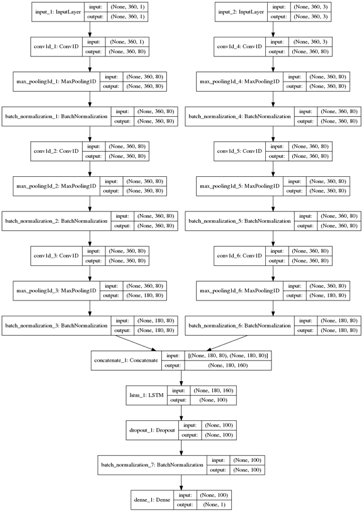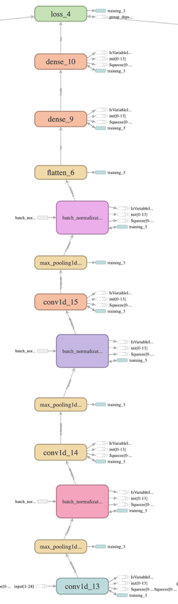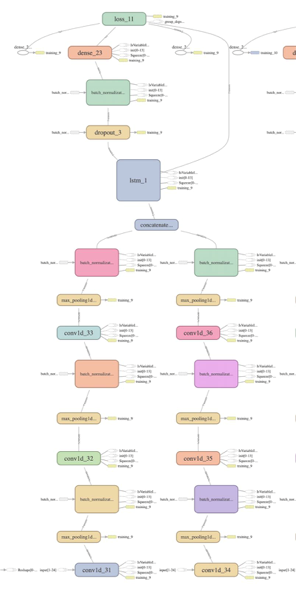2.3 提高模型的训练效果
在模型搭建中，发现在训练epochs比较大的时候使用Adam优化器能够比SGD优化器带来更好的效果，误差减小的速度比SGD更快，在卷积层后面加入batch normalization层后，训练的速度也有显著的提升，在实验的过程中一开始模型训练epochs还很小的时候就出现了在训练样本集合中误差随着优化的进行不断减小，而验证集的误差却有增大的迹象，说明模型开始出现过拟合，这时在卷积核和LSTM细胞中加入了L1正则化项，并且把卷积核大小增加，LSTM细胞隐含层变量的维度也调大，还在最后输出神经元之前加入Dropout和Early stopping机制，再次重新训练，模型过拟合的现象得到缓解。
代价函数的值在训练集上的变化曲线：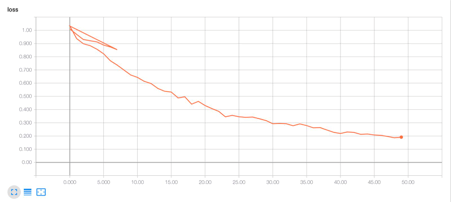测试集代价函数值变化情况三. 模型结果
该量化交易策略主要侧重价格的预测，尝试使用更加合理的模型对价格波动进行解释及预测，在交易策略部分使用了比较简单的设置，即在模型训练好后，在预测上涨的可能性较大的时候下多单，或者在模型预测的下跌可能性较大的时候下空单，之后持有10个单位时间，并设有止损机制，若在10个单位时间之前没有达到止损线就不平仓，持有时间达到10个单位时间后再平仓，反之，提前平仓。
下图是在20180902-20190425这段时间在螺纹钢期货上回测的净值曲线：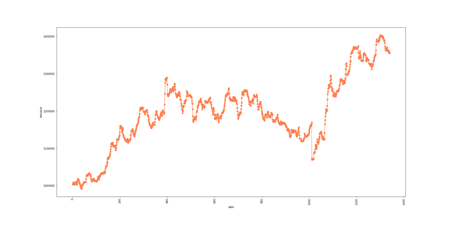可以看到一开始净值为1000000，到了回测结束的时候有1370000左右，回测期间收益达到百分之37%，并且期间的最大回撤也处于可以接受的范围，总体预测准确度达到百分之61.2%，在新数据集上的表现比前面所讲的三种对比模型都要好，且更稳定，实证了模型的有效性。由于本文主要侧重于预测模型的搭建，选用的数据只包含开盘价，收盘价，最高价，最低价，成交量这五个特征，如果需要进一步研究的话，可以在数据方面加入更多特征，来支持模型学习到更多的规则。


展开全文机器学习
• [方法/过程] 提出一种基于机器学习算法的研究热点趋势预测方法与分析框架，以基因工程领 域为例利用主题概率模型识别 WOS 核心集中论文摘要数据研究热点主题并进行主题演化关联构建，然后选取 BP 神经网络、支持向量...机器学习
• 以人脸图像视觉识别为研究对象，研究了基于LSTM长短期记忆单元网络视觉识别算法，通过使用卷积神经网络学习人脸图像特征信息，然后使用LSTM网络建立序列知识，并生成描述性句子，作为特征序列，建立一种基于...
基于LSTM网络的视觉识别研究与实现详细版订阅本博客

https://blog.csdn.net/ccsss22/article/details/115429083

1.问题描述：

以人脸图像的视觉识别为研究对象，研究了基于LSTM长短期记忆单元网络的视觉识别算法，通过使用卷积神经网络学习人脸图像的特征信息，然后使用LSTM网络建立序列知识，并生成描述性的句子，作为特征序列，建立一种基于句子描述的LSTM网络的视觉识别算法，最后通过MATLAB对该算法进行了仿真验证，对于不同姿态，不同干扰因素影响下的目标图像，本文所提出的基于LSTM的视觉识别正确率可以达到76%以上。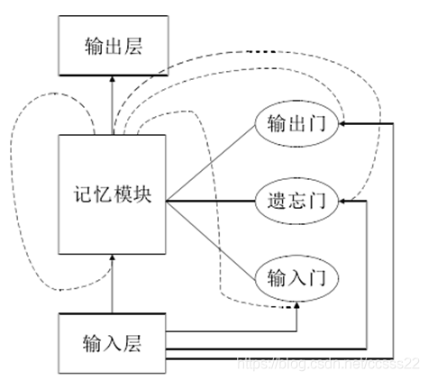2.部分程序：

function nn = func_LSTM(train_x,train_y,test_x,test_y);

binary_dim     = 8;
largest_number = 2^binary_dim - 1;
binary         = cell(largest_number, 1);

for i = 1:largest_number + 1
binary{i}      = dec2bin(i-1, binary_dim);
int2binary{i}  = binary{i};
end

%input variables
alpha      = 0.000001;
input_dim  = 2;
hidden_dim = 32;
output_dim = 1;

%initialize neural network weights
%in_gate = sigmoid(X(t) * U_i + H(t-1) * W_i)
U_i        = 2 * rand(input_dim, hidden_dim) - 1;
W_i        = 2 * rand(hidden_dim, hidden_dim) - 1;
U_i_update = zeros(size(U_i));
W_i_update = zeros(size(W_i));

%forget_gate = sigmoid(X(t) * U_f + H(t-1) * W_f)
U_f        = 2 * rand(input_dim, hidden_dim) - 1;
W_f        = 2 * rand(hidden_dim, hidden_dim) - 1;
U_f_update = zeros(size(U_f));
W_f_update = zeros(size(W_f));

%out_gate    = sigmoid(X(t) * U_o + H(t-1) * W_o)
U_o = 2 * rand(input_dim, hidden_dim) - 1;
W_o = 2 * rand(hidden_dim, hidden_dim) - 1;
U_o_update = zeros(size(U_o));
W_o_update = zeros(size(W_o));

%g_gate      = tanh(X(t) * U_g + H(t-1) * W_g)
U_g = 2 * rand(input_dim, hidden_dim) - 1;
W_g = 2 * rand(hidden_dim, hidden_dim) - 1;
U_g_update = zeros(size(U_g));
W_g_update = zeros(size(W_g));

out_para = 2 * zeros(hidden_dim, output_dim) ;
out_para_update = zeros(size(out_para));
% C(t) = C(t-1) .* forget_gate + g_gate .* in_gate
% S(t) = tanh(C(t)) .* out_gate
% Out  = sigmoid(S(t) * out_para)

%train
iter = 9999; % training iterations
for j = 1:iter

% generate a simple addition problem (a + b = c)
a_int = randi(round(largest_number/2));   % int version
a     = int2binary{a_int+1};              % binary encoding

b_int = randi(floor(largest_number/2));   % int version
b     = int2binary{b_int+1};              % binary encoding

% true answer
c_int = a_int + b_int;                    % int version
c     = int2binary{c_int+1};              % binary encoding

% where we'll store our best guess (binary encoded)
d     = zeros(size(c));

% total error
overallError = 0;

% difference in output layer, i.e., (target - out)
output_deltas = [];

% values of hidden layer, i.e., S(t)
hidden_layer_values = [];
cell_gate_values    = [];
% initialize S(0) as a zero-vector
hidden_layer_values = [hidden_layer_values; zeros(1, hidden_dim)];
cell_gate_values    = [cell_gate_values; zeros(1, hidden_dim)];

% initialize memory gate
% hidden layer
H = [];
H = [H; zeros(1, hidden_dim)];
% cell gate
C = [];
C = [C; zeros(1, hidden_dim)];
% in gate
I = [];
% forget gate
F = [];
% out gate
O = [];
% g gate
G = [];

% start to process a sequence, i.e., a forward pass
% Note: the output of a LSTM cell is the hidden_layer, and you need to
for position = 0:binary_dim-1
% X ------> input, size: 1 x input_dim
X = [a(binary_dim - position)-'0' b(binary_dim - position)-'0'];
% y ------> label, size: 1 x output_dim
y = [c(binary_dim - position)-'0']';
% use equations (1)-(7) in a forward pass. here we do not use bias
in_gate     = sigmoid(X * U_i + H(end, :) * W_i);  % equation (1)
forget_gate = sigmoid(X * U_f + H(end, :) * W_f);  % equation (2)
out_gate    = sigmoid(X * U_o + H(end, :) * W_o);  % equation (3)
g_gate      = tanh(X * U_g + H(end, :) * W_g);    % equation (4)
C_t         = C(end, :) .* forget_gate + g_gate .* in_gate;    % equation (5)
H_t         = tanh(C_t) .* out_gate;                          % equation (6)

% store these memory gates
I = [I; in_gate];
F = [F; forget_gate];
O = [O; out_gate];
G = [G; g_gate];
C = [C; C_t];
H = [H; H_t];

% compute predict output
pred_out = sigmoid(H_t * out_para);

% compute error in output layer
output_error = y - pred_out;

% compute difference in output layer using derivative
% output_diff = output_error * sigmoid_output_to_derivative(pred_out);
output_deltas = [output_deltas; output_error];

% compute total error
overallError = overallError + abs(output_error(1));

% decode estimate so we can print it out
d(binary_dim - position) = round(pred_out);
end

% from the last LSTM cell, you need a initial hidden layer difference
future_H_diff = zeros(1, hidden_dim);

% stare back-propagation, i.e., a backward pass
% the goal is to compute differences and use them to update weights
% start from the last LSTM cell
for position = 0:binary_dim-1
X = [a(position+1)-'0' b(position+1)-'0'];
% hidden layer
H_t = H(end-position, :);         % H(t)
% previous hidden layer
H_t_1 = H(end-position-1, :);     % H(t-1)
C_t = C(end-position, :);         % C(t)
C_t_1 = C(end-position-1, :);     % C(t-1)
O_t = O(end-position, :);
F_t = F(end-position, :);
G_t = G(end-position, :);
I_t = I(end-position, :);

% output layer difference
output_diff = output_deltas(end-position, :);
%         H_t_diff = (future_H_diff * (W_i' + W_o' + W_f' + W_g') + output_diff * out_para') ...
%                    .* sigmoid_output_to_derivative(H_t);

%         H_t_diff = output_diff * (out_para') .* sigmoid_output_to_derivative(H_t);
H_t_diff = output_diff * (out_para') .* sigmoid_output_to_derivative(H_t);

%         out_para_diff = output_diff * (H_t) * sigmoid_output_to_derivative(out_para);
out_para_diff =  (H_t') * output_diff;

% out_gate diference
O_t_diff = H_t_diff .* tanh(C_t) .* sigmoid_output_to_derivative(O_t);

% C_t difference
C_t_diff = H_t_diff .* O_t .* tan_h_output_to_derivative(C_t);

% forget_gate_diffeence
F_t_diff = C_t_diff .* C_t_1 .* sigmoid_output_to_derivative(F_t);

% in_gate difference
I_t_diff = C_t_diff .* G_t .* sigmoid_output_to_derivative(I_t);

% g_gate difference
G_t_diff = C_t_diff .* I_t .* tan_h_output_to_derivative(G_t);

% differences of U_i and W_i
U_i_diff =  X' * I_t_diff .* sigmoid_output_to_derivative(U_i);
W_i_diff =  (H_t_1)' * I_t_diff .* sigmoid_output_to_derivative(W_i);

% differences of U_o and W_o
U_o_diff = X' * O_t_diff .* sigmoid_output_to_derivative(U_o);
W_o_diff = (H_t_1)' * O_t_diff .* sigmoid_output_to_derivative(W_o);

% differences of U_o and W_o
U_f_diff = X' * F_t_diff .* sigmoid_output_to_derivative(U_f);
W_f_diff = (H_t_1)' * F_t_diff .* sigmoid_output_to_derivative(W_f);

% differences of U_o and W_o
U_g_diff = X' * G_t_diff .* tan_h_output_to_derivative(U_g);
W_g_diff = (H_t_1)' * G_t_diff .* tan_h_output_to_derivative(W_g);

% update
U_i_update = U_i_update + U_i_diff;
W_i_update = W_i_update + W_i_diff;
U_o_update = U_o_update + U_o_diff;
W_o_update = W_o_update + W_o_diff;
U_f_update = U_f_update + U_f_diff;
W_f_update = W_f_update + W_f_diff;
U_g_update = U_g_update + U_g_diff;
W_g_update = W_g_update + W_g_diff;
out_para_update = out_para_update + out_para_diff;
end

U_i = U_i + U_i_update * alpha;
W_i = W_i + W_i_update * alpha;
U_o = U_o + U_o_update * alpha;
W_o = W_o + W_o_update * alpha;
U_f = U_f + U_f_update * alpha;
W_f = W_f + W_f_update * alpha;
U_g = U_g + U_g_update * alpha;
W_g = W_g + W_g_update * alpha;
out_para = out_para + out_para_update * alpha;

U_i_update = U_i_update * 0;
W_i_update = W_i_update * 0;
U_o_update = U_o_update * 0;
W_o_update = W_o_update * 0;
U_f_update = U_f_update * 0;
W_f_update = W_f_update * 0;
U_g_update = U_g_update * 0;
W_g_update = W_g_update * 0;
out_para_update = out_para_update * 0;

end
————————————————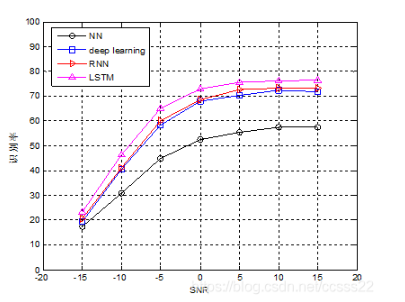A-05-40

展开全文• 黑色系统是指一个系统内部信息对外界来说是一无所知，只能通过它与外界联系来加以观测研究。 灰色系统内一部分信息是已知，另一部分信息是未知，系统内各因素间有不确定关系。 2 算法原理 二、源...
一、简介
LSTM
LSTM网络
long short term memory，即我们所称呼的LSTM，是为了解决长期以来问题而专门设计出来的，所有的RNN都具有一种重复神经网络模块的链式形式。在标准RNN中，这个重复的结构模块只有一个非常简单的结构，例如一个tanh层。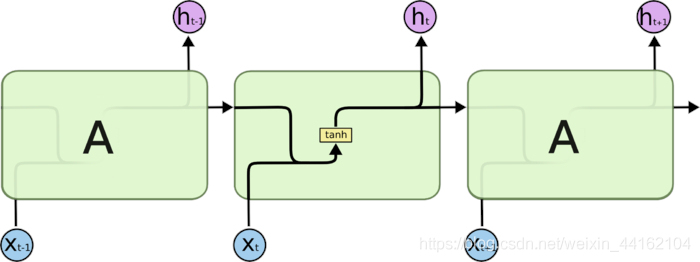图3.RNNcell
LSTM 同样是这样的结构，但是重复的模块拥有一个不同的结构。不同于单一神经网络层，这里是有四个，以一种非常特殊的方式进行交互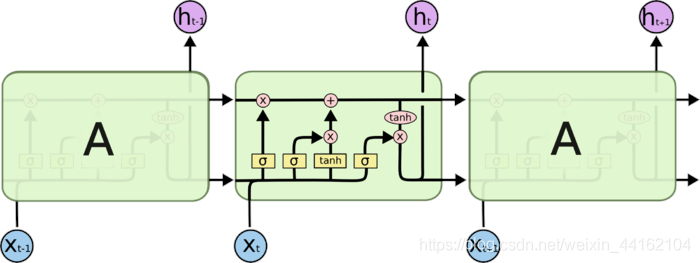图4.LSTMcell
LSTM核心思想
LSTM的关键在于细胞的状态整个(绿色的图表示的是一个cell)，和穿过细胞的那条水平线。
细胞状态类似于传送带。直接在整个链上运行，只有一些少量的线性交互。信息在上面流传保持不变会很容易。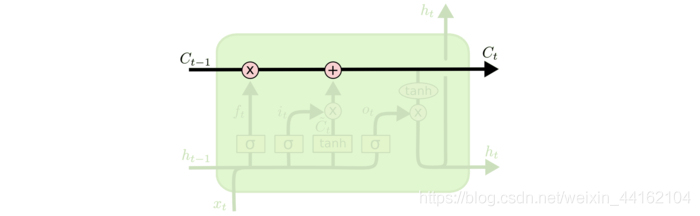图5.LSTMcell内部结构图
若只有上面的那条水平线是没办法实现添加或者删除信息的。而是通过一种叫做 门（gates） 的结构来实现的。
门 可以实现选择性地让信息通过，主要是通过一个 sigmoid 的神经层 和一个逐点相乘的操作来实现的。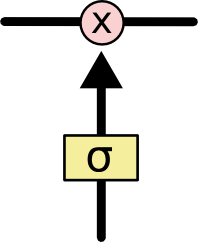图6.信息节点
sigmoid 层输出（是一个向量）的每个元素都是一个在 0 和 1 之间的实数，表示让对应信息通过的权重（或者占比）。比如， 0 表示“不让任何信息通过”， 1 表示“让所有信息通过”。
LSTM通过三个这样的基本结构来实现信息的保护和控制。这三个门分别输入门、遗忘门和输出门。
深入理解LSTM
遗忘门
在我们 LSTM 中的第一步是决定我们会从细胞状态中丢弃什么信息。这个决定通过一个称为忘记门层完成。该门会读取h t − 1 h_{t−1}ht−1​和x t x_txt​，输出一个在 0到 1之间的数值给每个在细胞状态C t − 1 C_{t-1}Ct−1​中的数字。1 表示“完全保留”，0 表示“完全舍弃”。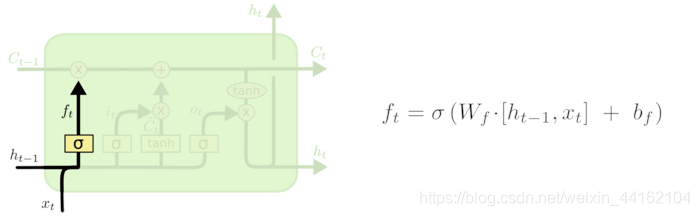其中ht−1表示的是上一个cell的输出，xt表示的是当前细胞的输入。σσ表示sigmod函数。
输入门
下一步是决定让多少新的信息加入到 cell 状态 中来。实现这个需要包括两个 步骤：首先，一个叫做“input gate layer ”的 sigmoid 层决定哪些信息需要更新；一个 tanh 层生成一个向量，也就是备选的用来更新的内容，C^t 。在下一步，我们把这两部分联合起来，对 cell 的状态进行一个更新。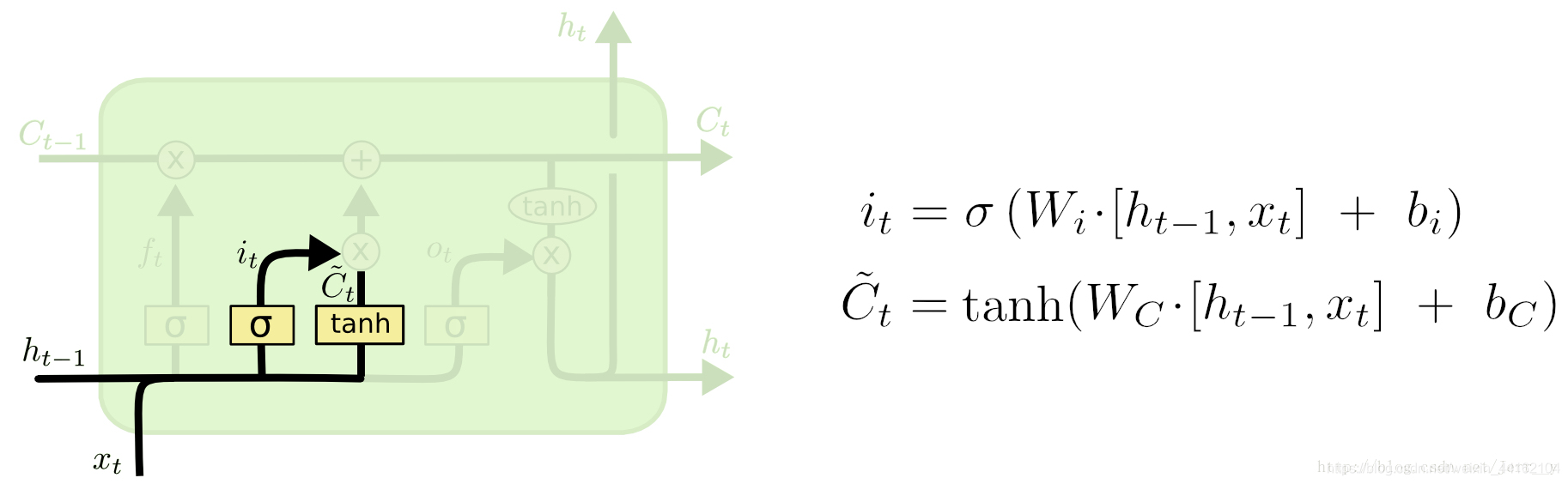现在是更新旧细胞状态的时间了，Ct−1更新为Ct。前面的步骤已经决定了将会做什么，我们现在就是实际去完成。
我们把旧状态与ft相乘，丢弃掉我们确定需要丢弃的信息。接着加上it∗C~t。这就是新的候选值，根据我们决定更新每个状态的程度进行变化。
输出门
最终，我们需要确定输出什么值。这个输出将会基于我们的细胞状态，但是也是一个过滤后的版本。首先，我们运行一个 sigmoid 层来确定细胞状态的哪个部分将输出出去。接着，我们把细胞状态通过 tanh 进行处理（得到一个在 -1 到 1 之间的值）并将它和 sigmoid 门的输出相乘，最终我们仅仅会输出我们确定输出的那部分。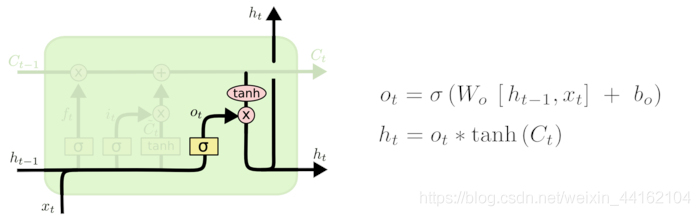二、源代码
%%
clc
clear all
close all
%加载数据，重构为行向量
num=100;
x=1:num;
db=0.1;
data =abs(0.5.*sin(x)+0.5.*cos(x)+db*rand(1,num));
data1 =data;%把你的负荷数据赋值给data变量就可以了。
%data是行向量。要是还不明白，就留言吧。

%%
%序列的前 90% 用于训练，后 10% 用于测试
numTimeStepsTrain = floor(0.9*numel(data));
dataTrain = data(1:numTimeStepsTrain+1);
dataTest = data1(numTimeStepsTrain+1:end);

%数据预处理，将训练数据标准化为具有零均值和单位方差。
mu = mean(dataTrain);
sig = std(dataTrain);
dataTrainStandardized = dataTrain;

%输入LSTM的时间序列交替一个时间步
XTrain = dataTrainStandardized(1:end-1);
YTrain = dataTrainStandardized(2:end);
%%
%创建LSTM回归网络，指定LSTM层的隐含单元个数96*3
%序列预测，因此，输入一维，输出一维
numFeatures = 1;
numResponses = 1;
numHiddenUnits = 20*3;

layers = [ ...
sequenceInputLayer(numFeatures)
lstmLayer(numHiddenUnits)
fullyConnectedLayer(numResponses)
regressionLayer];
%% WOA
lb=0.001;%学习率下限
ub=0.1;%学习率上限

% Main loop

while t<Max_iter
t

end

%将预测值与测试数据进行比较。
figure(1)
subplot(2,1,1)
plot(YTest,'gs-','LineWidth',2)
hold on
plot(YPred_best,'ro-','LineWidth',2)
hold off
legend('观测值','预测值')
xlabel('时间')
ylabel('数据值')
title('Forecast with Updates')

subplot(2,1,2)
stem(YPred_best - YTest)
xlabel('时间')
ylabel('均方差值')
title('均方差图 ' )

figure(2)
plot(dataTrain(1:end-1))
hold on
idx = numTimeStepsTrain:(numTimeStepsTrain+numTimeStepsTest);
plot(idx,[data(numTimeStepsTrain) YPred_best],'.-')
hold off
xlabel('时间')
ylabel('数据值')
title('预测图')
legend('观测值', '预测值')

figure(3)
plot(1:Max_iter,Convergence_curve,'bo-');
hold on;
title('鲸鱼优化后Error-Cost曲线图');
xlabel('迭代次数')
ylabel('误差适应度值')

三、运行结果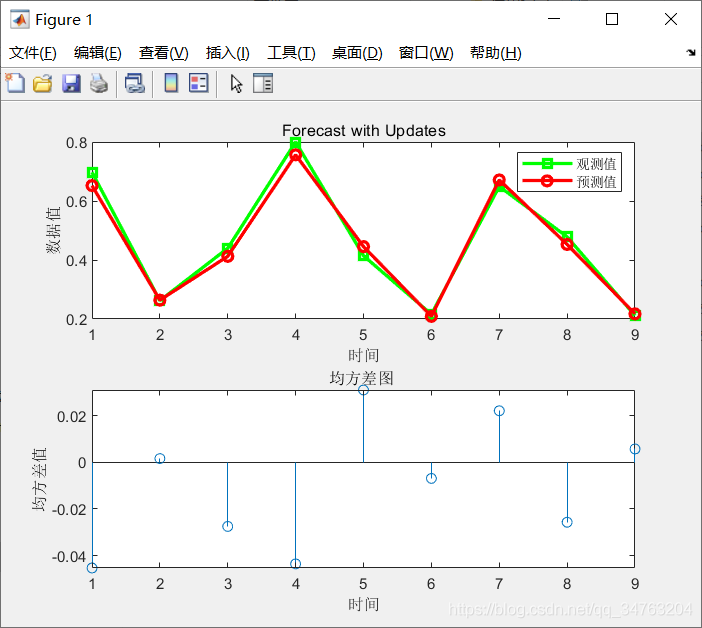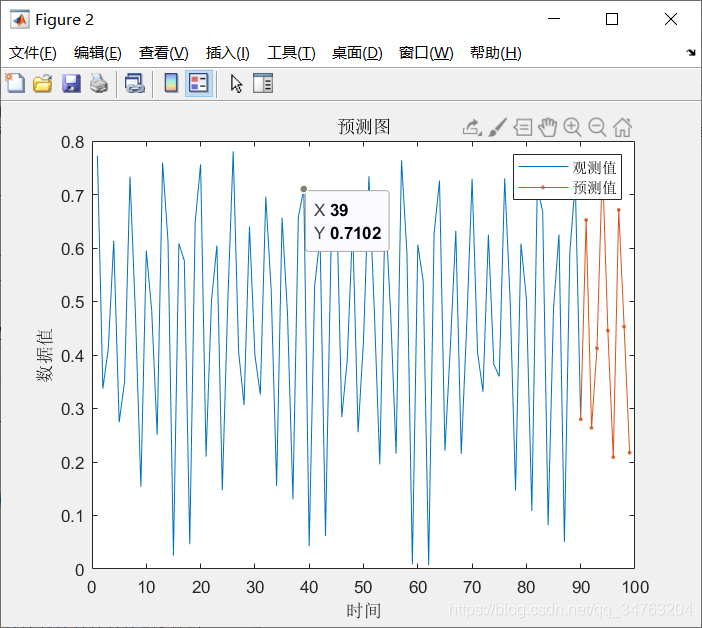四、备注
版本：2014a


展开全文• 这项研究旨在研究和实现一种人工智能（AI）算法，该算法将实时分析音频文件，识别并呈现其中表达情感。 此分类模型是通过深度学习方法开发，这意味着深度神经网络（DNN），同时选择了用于时间序列分析高级...
• 点击率预测是计算广告学核心算法之一。传统浅层模型没有充分考虑到数据之间存在...实验结果证明：与浅层模型或单一结构神经网络模型相比，基于卷积-LSTM的混合神经网络模型能有效提高广告点击事件预测准确率。
• 然后，检测模型根据光伏阵列布局特点设计了一种特征提取算法，分别提取光伏阵列电流横向与纵向特征，来获取空间与时间上特性；再通过CNN网络来对横向特征做进一步提取与纵向特征压缩，以解决特征种类单一及...
• 研究目的 进行预测餐饮中每天菜品销量，提前制定好采购计划，减小库存，提高预算。 分析思路 1.销量异常日展示 2.最冷款慢销菜品展示（可降价） 目的：提升销量 3.最优畅销菜涨价名称展示（可涨价） 目的：提升...
研究目的
进行预测餐饮中每天菜品的销量，提前制定好采购计划，减小库存，提高预算。
分析思路
1.销量异常日展示
2.最冷款慢销菜品展示（可降价）   目的：提升销量
3.最优畅销菜涨价名称展示（可涨价）  目的：提升利润
4.菜品套餐营销组合价格策略（畅销菜涨价+滞销菜降价）*0.95%  目的：促销量
5.菜品偏好相关展示（目的：推送。由于买A菜的人，买了B菜，还买了C菜。推荐下一个有买A菜的人，也尝试C菜）
以一周的销量为主，对每周的销售总量进行预测，对菜在这一周的总销量进行拆解，均分到每一天，了解每一天菜的销量。
实施过程
部分数据如下：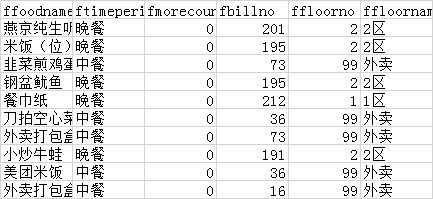分析程序算法如下：
import matplotlib
import pandas as pd
from sklearn import preprocessing
import numpy as np
from sklearn.preprocessing import Imputer
import matplotlib.pyplot as plt
import warnings
from datetime import datetime
import xlrd
import time
warnings.filterwarnings(“ignore”)
matplotlib.rcParams[‘font.sans-serif’] = [‘FangSong’] # 指定默认字体
matplotlib.rcParams[‘axes.unicode_minus’] = False # 解决保存图像是负号’-'显示为方块的问题
data=pd.read_csv(’/Users/wang/PycharmProjects/untitled2/15650711345953342991000.csv’)#读取数据
df=data[[‘fshopday’,‘ffoodname’,‘fsubsaleqty’]]#去除无关信息
foodname_set=df[‘ffoodname’].unique().tolist()#探寻食品种类
len(foodname_set)#食物种类数量
group_obj = df.groupby(‘ffoodname’)#通过ffoodname键分组
dis_data=group_obj.size()#查看每种食品的非空项数量
dis_data.describe()#非空数量统计
dis_data.name=‘ffoodname_box’
dis_data=dis_data/138 #计算1-缺失率，即非空率
dis_data.plot.box()#非空率箱型图
#通过访问分组信息，按照时间为索引，连接数据
new_cols = pd.DataFrame()
count = 0
for name, group in group_obj:
group = group[[‘fshopday’, ‘fsubsaleqty’]]
group.rename(inplace=True, columns={“fsubsaleqty”: name})#重命名
count += 1
if count == 1:
new_cols = group
else:
new_cols = pd.merge(new_cols, group, how='outer', on='fshopday')#连接新的一天数据与之前的

print('正在拼接第{}个，总共178个'.format(count))

new_cols[‘fshopday’] = pd.to_datetime(new_cols[‘fshopday’])#时间转为datetime
new_cols.index = new_cols[‘fshopday’]#时间变成索引
del new_cols[‘fshopday’]#删除这列
new_cols=new_cols.sort_index()#根据时间排序
copy_cols=new_cols.copy()
#观察缺失值分布图
def get_miss_info(data):
m,n=data.shape
miss_data=np.zeros((m,n))
row_list=[]
col_list=[]
indexs=list(data.index)
columns=list(data.columns)
null_data=data.isnull()
for i in range(m):
for j in range(n):
if(null_data.iloc[i,j]==True):
miss_data[i,j]=1
row_list.append(i)
col_list.append(j)
#plt.figure(figsize=(20, 18))
#plt.scatter(col_list,row_list)
#plt.show()
#get_miss_info(new_cols)
#plt.savefig(‘all_miss.png’)
isnull_rate=new_cols.apply(lambda col:sum(col.isnull())/col.size)#得到缺失率数据
cut_rate=0.5#只保留缺失值小于cut_rate的列名
delete_col_names=list(isnull_rate[isnull_rate>cut_rate].index)#得到缺失率高的列名
new_cols.drop(columns=delete_col_names,inplace=True)#去掉缺失高的列
get_miss_info(new_cols)
#去掉近期的缺失值
isnull_rate=new_cols.iloc[-20:].apply(lambda col:sum(col.isnull())/col.size)#得到缺失率数据
cut_rate=0.3#只保留缺失值小于cut_rate的列名
delete_col_names=list(isnull_rate[isnull_rate>cut_rate].index)#得到缺失率高的列名
new_cols.drop(columns=delete_col_names,inplace=True)#去掉缺失高的列
get_miss_info(new_cols)
#填充缺失值,因为数据是时间序列，采用插值填充，使用前面数据填充后面的，剩下的最前面的缺失值，使用中值填充
#new_cols.fillna(method=‘time’,inplace=True)#先向前填充
col_names=new_cols.columns
for col_name in col_names:
new_cols[col_name]= new_cols[col_name].interpolate(method=‘linear’)
new_cols.fillna(new_cols.median(),inplace=True)#再中位数填充
#最后七天的真实值
true_cols=new_cols.iloc[-7:,:].copy()
#处理异常值
#异常值判断标准x > Q3 + k(Q3 - Q1) OR
#x < Q1 - k(Q3 - Q1)
#k是自己定义的参数
k=5
m=5#使用前m个数进行移动平均
def get_outlier():
describe = new_cols.describe()
count=0
for row in range(new_cols.shape):
for col in range(new_cols.shape):
if (new_cols.iloc[row, col] > (
describe.iloc[6, col] + k * (describe.iloc[6, col] - describe.iloc[4, col]))):#若数太小
print(‘异常值为{}日{}菜种，异常数量{}’.format(new_cols.index[row],new_cols.columns[col],new_cols.iloc[row,col]))
if(row>=m):#若数据不在开头m行和
temp=0
for j in range(1,m+1):#滑动到前几个数据，取平均
temp=temp+new_cols.iloc[row-m,col]
new_cols.iloc[row,col]=temp/m
else:
new_cols.iloc[row,col]=new_cols.iloc[row-1,col]
count=count+1
if( new_cols.iloc[row, col] < (
describe.iloc[4, col] - k * (describe.iloc[6, col] - describe.iloc[4, col]))):
print(‘异常值为{}日{}菜种，异常数量{}’.format(new_cols.index[row],new_cols.columns[col],new_cols.iloc[row,col]))
if( row>=m):#若数据不在开头m行和
temp=0
for j in range(1,m+1):#滑动到前几个数据，取平均
temp=temp+new_cols.iloc[row-m,col]
new_cols.iloc[row,col]=temp/m
else:
new_cols.iloc[row,col]=new_cols.iloc[row-1,col]
count=count+1
return count
print(‘异常值数量：{}’.format(get_outlier()))
#作图分析，探究时间与分布的关系
names=[‘一鸡两吃’,‘下饭姜爆鸡’,‘下饭扑四脆’,‘鲜啤500ml’]
new_cols[names].plot()
#plt.savefig(‘dis.png’)
def is_weenkend(data):
temp=data.weekday()#获得一周第几天，0代表周一，6代表星期天
if(temp>=5):
weekend=1
else:
weekend=0
return weekend
rest_holiday=[
‘2018-12-31’,
‘2019-01-01’,‘2019-02-04’,‘2019-02-05’,‘2019-02-06’,‘2019-02-07’,‘2019-02-08’,
‘2019-04-05’,‘2019-04-29’,‘2019-04-30’,‘2019-05-01’,‘2019-06-07’,‘2019-09-13’,
‘2019-10-01’,‘2019-10-02’,‘2019-10-03’,‘2019-10-04’,‘2019-10-07’,‘2019-12-30’,
‘2019-12-31’,
‘2020-01-01’,‘2020-01-24’,‘2020-01-27’,‘2020-01-28’,‘2020-01-29’,‘2020-01-30’,
‘2020-04-06’,‘2020-05-01’,‘2020-06-25’,‘2020-06-26’,‘2020-10-01’,‘2020-10-02’,
‘2020-10-05’,‘2020-10-06’,‘2020-10-07’,‘2020-10-08’,
‘2021-01-01’,
]
def is_holiday(data):#获得节假日
set_date_str=data.strftime(’%Y-%m-%d’)
if set_date_str in rest_holiday:
is_holiday=1
else:
is_holiday=0
return is_holiday
def get_month(data):#获得月份
month=data.month
if(month==8):
month=7
return str(month)
def get_searon(data):
month=data.month
if(month  in ):
label=‘1’
elif(month  in [3,4,5]):
label=‘2’
else:
label=‘3’
return label
def get_weekday(data):
temp=data.weekday()
return str(temp)
#gghj
def delete_week(text):
temp=[]
for a in text:
if( a.isdigit() or a in [’-’]):
temp.append(a)
text=’’.join(temp)
return text
def get_wendu(text):
return int(text[:-1])
def get_weather(text):
w=[]
for a in text:
if(a in [‘雨’]):
w.append(0)
elif(a in [‘阴’]):
w.append(1)
else:
w.append(2)
if(len(w)0):
return ‘2’
else:
return str(min(w))
def get_wind(text):
return str(int(text[-2]))
def get_poution(text):
if(text[-1]‘优’):
return ‘0’
elif(text[-1]==‘良’):
return ‘1’
else:
return ‘2’
weather=pd.read_excel(’/Users/wang/PycharmProjects/untitled2/weather.xlsx’)
weather[‘日期’]=weather[‘日期’].map(delete_week)
weather[‘日期’] = pd.to_datetime(weather[‘日期’])#时间转为datetime
weather.index = weather[‘日期’]#时间变成索引
weather.index.name=‘date’
new_cols=pd.merge(new_cols, weather, how=‘inner’, left_on=‘fshopday’,right_on=‘日期’)
new_cols.index = new_cols[‘日期’]#时间变成索引
del new_cols[‘日期’]#删除这列
new_cols=new_cols.sort_index()#根据时间排序
new_cols.head()
new_cols[‘is_weekend’]=0
new_cols[‘is_holiday’]=0
new_cols[‘month’]=‘a’
new_cols[‘seaon’]=‘a’
new_cols[‘weekday’]=‘a’
#将是否周末，月份，节假日作为特征，写入dataframe
for row in new_cols.index:
new_cols.loc[row,‘is_weekend’]=is_weenkend(row)#得到周末特征
new_cols.loc[row,‘is_holiday’]=is_holiday(row)#得到节假日特征
new_cols.loc[row,‘month’]=get_month(row)#得到月份特征
new_cols.loc[row,‘seaon’]=get_searon(row)
new_cols.loc[row,‘weekday’]=get_weekday(row)
new_cols[‘天气’]=new_cols[‘天气’].map(get_weather)
new_cols[‘风力’]=new_cols[‘风力’].map(get_wind)
new_cols[‘高温’]=new_cols[‘高温’].map(get_wendu)
new_cols[‘低温’]=new_cols[‘低温’].map(get_wendu)
new_cols[‘污染指数’]=new_cols[‘污染指数’].map(get_poution)
new_cols=pd.get_dummies(new_cols)#对月份进行one-hot编码
dummies_num=26
new_cols.head()
#对数据进行归一化
from sklearn.preprocessing import MinMaxScaler,StandardScaler,RobustScaler
scale=MinMaxScaler()#最大最小归一化
feature_1=scale.fit_transform(new_cols.iloc[:,:-dummies_num])#除了周末，节假日，月份，其他特征进行缩放
all_features=np.hstack((feature_1,new_cols.iloc[:,-dummies_num:].values))#连接所有特征
#制作训练集
day_num=7
split_num=80
#train_data=np.zeros((split_num,day_num,all_features.shape))#训练集数据，可以理解为前7天数据
test_data=np.zeros((split_num,day_num,all_features.shape))
test_label=np.zeros((split_num,7,all_features.shape-dummies_num-2))
#train_label=np.zeros((split_num,7,all_features.shape-dummies_num-2))#训练集标签，可以理解为未来7天数据
train_data=np.zeros((feature_1.shape-day_num-split_num-7-7,day_num,all_features.shape))#测试集数据
train_label=np.zeros((feature_1.shape-day_num-split_num-7-7,7,all_features.shape-dummies_num-2))#测试集标签
for i in range(split_num-20,split_num):#遍历all_features前100行，用i:i+7作为训练数据，i+7:i+14预测
test_data[i,:,:]=all_features[i:i+day_num,:]
test_label[i,:,:]=all_features[i+7:i+14,:(-dummies_num-2)]
test_data[0,:,:]=all_features[-7-day_num:-7,:]
test_label[0,:,:]=all_features[-7:?-dummies_num-2)]
for i in range(split_num,(new_cols.shape-7-day_num-7)):#遍历all_features80行以后的，用i:i+7作为训练数据，i+7:i+14预测。-7为了不用最后7天数据
train_data[i-split_num,:,:]=all_features[i:i+day_num,:]
train_label[i-split_num,:,:]=all_features[i+7:i+14,:(-dummies_num-2)]
#搭建lstm模型
from keras.layers import LSTM,Dense,RepeatVector,TimeDistributed
from keras.models import Sequential,load_model
from keras.callbacks import ModelCheckpoint
from numpy.random import seed
seed(1)
n_steps_in, n_steps_out = day_num, 7#输入时间轴7天，输出也是7天
n_features = all_features.shape# 此例中 n features = 特征数量，
#搭建一个两层的lstm网络
model = Sequential()
model.add(LSTM(32, activation=‘relu’, input_shape=(n_steps_in, n_features)))#第一层lstm网络
model.add(RepeatVector(n_steps_out))#
model.add(LSTM(16, activation=‘relu’, return_sequences=True))#第二层lstm网络
model.add(TimeDistributed(Dense(n_features-dummies_num-2)))#输出特征数量
model.compile(optimizer=‘adam’, loss=‘mse’)#损失函数使用mse，均值损失。优化器使用adam。
‘’’
model = Sequential()
model.add(LSTM(32, activation=‘relu’, input_shape=(n_steps_in, n_features),return_sequences=True))#第一层lstm网络
model.add(LSTM(n_features-dummies_num-2, activation=‘relu’, return_sequences=True))#第二层lstm网络
model.compile(optimizer=‘adam’, loss=‘mse’)
‘’’
filepath = “best_weights.h5”
checkpoint = ModelCheckpoint(filepath, monitor=‘val_loss’, verbose=0, save_best_only=True, mode=‘min’)
callbacks_list = [checkpoint]
model.summary()#模型结构，每一层的输出数量。参数数量
samples=np.zeros((train_data.shape,))
#模型权重
temp=0.1
for i in range(samples.shape):
temp=temp+0.1
samples[i]=0.1+temp
history=model.fit(train_data,train_label,epochs=100,batch_size=8,validation_data=(test_data,test_label),sample_weight=samples,callbacks=callbacks_list)#模型训练过程
#画出模型训练和测试孙树图像
#plt.clf()
#plt.plot(history.history[‘val_loss’])
#plt.plot(history.history[‘loss’])
#plt.legend([‘val_loss’, ‘loss’], loc=‘upper left’)
#plt.savefig(‘plot_loss.png’)
model=load_model(‘best_weights.h5’)
#取出最后7天数据，预测未来7天
laster_7days=all_features[-(7+day_num):-7]#取出数据
laster_7days=laster_7days.reshape((1,day_num,-1))#转换数据格式
future_7days=model.predict(laster_7days)#预测未来7天
temp=future_7days
future_7days=np.hstack((temp,np.ones((7,2))))
pre_no_scare=scale.inverse_transform(future_7days)
#pre_no_scare=scale.inverse_transform(future_7days[:,:-7])#将数据还原为缩放前
pre=pre_no_scare[:,:-2]
pre=pre.round()#四舍五入结果
#将结果写入dataframe，并且存储结果到csv
dates=[datetime(2019,8,8),datetime(2019,8,9),datetime(2019,8,10),
datetime(2019,8,11),datetime(2019,8,12),datetime(2019,8,13),datetime(2019,8,14)]
data_pre=pd.DataFrame(pre,columns=new_cols.columns[?-dummies_num-2)],index=dates)
data_pre.index,name
cols_list=list(new_cols.columns[?-dummies_num-2)])
data_pre.index.name=‘time’
new_cols.index.name=‘time’
data_out=pd.concat([new_cols[cols_list],data_pre])
print(data_out)
data_out.to_csv(’/Users/wang/PycharmProjects/untitled2/data_out.csv’)


展开全文时间序列 递归神经网络
• 针对群体情绪识别准确率问题，结合卷积神经网络（CNN）与长短期记忆网络...实验结果表明，模型可有效地识别四种典型群体情绪，且识别率高于已有算法，准确度（ACC）和宏平均精度（MAP）分别最高可达82.6%、84.1%。卷积神经网络 长短期记忆网络
• 本文的安装环境为tensorflow2.1，使用的算法为LSTM算法，数据集为keras的imdb数据集。对于研究tensorflow2.1中LSTM算法的人可以借鉴参考
• 以人脸图像视觉识别为研究对象，研究了基于LSTM长短期记忆单元网络视觉识别算法，通过使用卷积神经网络学习人脸图像特征信息，然后使用LSTM网络建立序列知识，并生成描述性句子，作为特征序列，建立一种...人脸识别 深度学习 机器学习
• 最近在做lip reading领域的研究，设计到C3D和RNN的结合，于是仔细观摩了下LSTM的系列论文，总结如下：PPT总长98页，内容包括： 1.conventional LSTM（含BPTT算法的详细讲解） 2.forget gate的提出 3.Peephole ...
• 在对容器云资源预测问题研究现状进行调查后，提出一种采用自适应概率多选择策略遗传算法（APMSSGA）优化长短期记忆网络（LSTM容器云资源预测模型。实验结果表明，与简单遗传算法（SGA）相比，APMSSGA在LSTM...容器云 长短期记忆网络
• 他们将阅读新闻，研究公司历史，行业趋势以及做出预测其他许多数据点。 流行理论是，股票价格是完全随机且不可预测，但这提出了一个问题，为什么摩根士丹利和花旗集团这样顶级公司会聘请定量分析师来建立...
• 机场是一个空地交通系统,机场地面交通预测不同于一般城市道路具有其特殊性。本研究以北京首都国际机场为研究对象,基于2016年8月1日-2017年...与线性模型ARIMA和VAR模型相比,深度学习算法LSTM模型具有更好预测精度。
• 该存储库源于Google研究人员赞助正在进行Kaggle竞赛-文本规范化。 对于TTS（文本到语音）系统，需要在将原始文本输入到系统之前对其进行规范化，以便可以从中生成语音。 例如，如果将文本“ 123”输入算法，则...Python
• 　一直想写一篇面对初学者文章从上层应用到底层机器学习基础方面全流程阐释当下流行深度学习算法（CNN，RNN等）。刚好春节前有同学工作内容涉及对话机器人，我也从零开始接触学习了下，所以就借这个案例展开...
• 采集煤矿正常生产期间的瓦斯监测数据作为训练数据,利用深度学习框架TensorFlow进行算法的仿真,并研究了不同时间步长、网络深度下的LSTM以及多信息融合对瓦斯预测模型性能的影响。实验结果在1 000条测试数据集上获得...
• 基于 LSTM POI 个性化推荐框架AbstractQuestionMethodModelIntroduction作者为什么研究这个课题?参考模型介绍① word2vec②CBOW模型③ LSTM长依赖存在问题LSTM 网络LSTMs核心思想一步一步理解LSTM相关工作与...神经网络
• 1 粒子群算法的概念 粒子群优化算法(PSO：Particle swarm optimization) 是一种进化计算技术（evolutionary computation）。源于对鸟群捕食的行为研究。粒子群优化算法的基本思想：是通过群体中个体之间的协作和信息...
• 这篇文章将详细讲解Keras实现经典深度学习文本分类算法，包括LSTM、BiLSTM、BiLSTM+Attention和CNN、TextCNN，这篇文章将以代码为主，让读者直观感受深度神经网络及对比实验。个人感觉还不错，基础性文章，希望对...
• 论文 技术分析《关于网络分层信息泄漏点快速检测仿真》 "1、基于动态阈值泄露点快速检测方法,采样Mallat算法对网络分层信息离散采样数据进行离散小波变换;利用滑动窗口对该尺度上小波系数进行加窗处理,计算...
• 重庆大学硕士学位论文 中文摘要 摘 要 交通预测问题是智能交通系统研究的重要问题交通速度是衡量交通状态 一个重要指标, 交通速度预测不仅可以为交通管理者提供科学依据还可以为其他 道路服务如路径规划提供支持...
• 粒子群算法是在1995年由Eberhart博士和Kennedy博士一起提出的，它源于对鸟群捕食行为的研究。它的基本核心是利用群体中的个体对信息的共享从而使整个群体的运动在问题求解空间中产生从无序到有序的演化过程，从而......